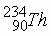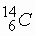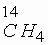CHEMISTRY

 Time: 1 Hour Max.Marks : 60

Instructions:

(1) Answer must be written either in English or the medium of instruction of the candidate in high school.

(2) There will be no negative marking

(3) Use of calculators or graph papers is not permitted

(4) There are FOUR questions. Answer all the questions.

I. Multiple choice. Each question has more than one answer. Indicate the correct answer by A,B,C,D. (10x1½=15 Marks)

1. All the elements in a group in the periodic table have different

A) atomic weights B) number of electrons C) mass number D) atomic number

2. To understand the behaviour of molecules it is necessary to have a thorough understanding of which of the following properties of chemical bonding

A) bond types B) bond angles C) bond lengths D) bond energies

3. Choose the correct statements

A) the density of a gas varies with temperature

B) the density of a gas varies with pressure

C) the volume of a gas always increases when temperature increases

D) the volume of a gas may not increase when the temperature increases

4. The photosynthesis reaction is 6CO2(g) + 6H2O(l) C6H12O6(s) + 6O2(g) with D H=2802KJ consider the reaction to be at equilibrium. In order to increase the synthesis of

C6H12O

A) the amount of CO2 should be increased

B) the amount of H2O should be increased

C) the temperature should be increased

D) the partial pressure of CO2 should be increased

5. Which of the following statements are correct for weak acid

A) only some of the ionisable hydrogen atoms are present as hydronium ions

B) the proton transfer equilibrium favours the ionised acid molecules

C) weakness refers to the number of moles of acid for litre

D) all simple carboxylic acids containing-COOH group are weak acids in water

6. Choose the correct alternatives

A) in all calculations involving solubility products the ion concentrations are expressed in units of solute per litre

B) in all calculations involving solubility products two ion concentrations are expressed in units of solute per litre of solution

C) molar solubility of a compound in a solution increases of the solution has common ion with the compound

D) molar solubility of a compound in a solution decreases if solution has a common ion with the compound

7. A zero order reaction is one in which

A) the rate of reaction is zero

B) the rate of the reaction is independent of concentrations

C) the power of the reactant in the rate law is zero

D) the rate of the reaction is equal to the rate constant k

8. According to the electro chemical series

A) metal above hydrogen are rarely found in free state

B) metals below hydrogen are often found in free state

C) highly electropositive metals occurs in association with non-metals

D) highly electro-negative metals occur in association with metalloids

9. Various metallurgical process employed for the extraction of metals depend on

A) the nature of the ore chosen for metal extraction

B) the type of the materials associated with ore

C) the percentage of the metal present in the ore

D) the degree of purity required in the extracted metal

10. Choose correct statements

A) the positive ions will be larger than their parent atoms

B) the positive ions will be smaller than their parent atoms

C) the negative ions will be larger than their parent

D) the negative ions will be smaller than their parent atoms

II. Fill in the blanks: (30x1 = 30 Marks)

1. NaCl on reaction with con H2SO4 produce HCl(g) similarly HBr(g) can be prepare by treating NaBr with ________ acid.

2. Physical state of the matter is function of temperature one state of the matter can be converted into another state. The favourable conditions for the conversions of a gas in to liquid, are _________ pressure __________ temperature.

3. Magnesium is extracted from molten MgCl2 by electrolytic reduction method. During this process coal gas is passed through the cell. This role of the coal gas is ________.

4.is radioactive element with a half life of 24 days. This is much shorter than the age of the earth. This isotope is still existing in the nature. Reason for the existence of the isotope is _________.

5. In fertilizer industry hydrogen gas is prepared from catalytic reaction represented by the equation CnH2n+2 + H2O ® CO2 + H2 for n=4. 429.12x1014 lit (STP) of H2 is daily produced. Daily consumption of hydrocarbon and steam are ___________ , _________.

6. A student is placed a pellet of NaOH in a watch glass. After some time it becomes liquidous and superficially thin solid layer is formed. The composition of the layer is _______ chemical equation of the reaction is ________.

7. On heating colourless solid A, a gas B (liquid at room temperature) and gas "C" are formed. A is also decomposed by NaOH on heating to form gas D. This gas D gives white fumes with HCl. Gas C occupies 800 ml/gram at STP. Chemical formula of A is ______.

8. It is an oxide of nitrogen which reacts with NaOH solution to produce sodium nitrate and sodium nitrite. The electronic formula of gas is ________.

9. The equilibrium constant for the reaction H2(g) + I2(g) 2HI is Ki and Kn for the reaction nH2(g) + nI2(g) 2nHI(g) . The relation ship between K1 and Kn is ________.

10. 0.76 grams of KOH completely neutralize 1 gram of a unknown acid the basicity of the acid is ________.

11. Equal weights of ethane and hydrogen are mixed in an empty container at 250C. The fraction of the total pressure exerted by H2 is ________.

12. Hydrogen halides HF, HCl, HBr, HI are used as the acids. The decreasing order of the strength of these acids is ________.

13.is a beta active nucleus. A sample ofgas kept in a closed vessel shows increase in pressure this is due to the ________.

14. Helium atom is two times heavier than hydrogen molecule. At 298 K the average kinetic energy of helium atom is ________ as that of hydrogen molecule.

15. Considerable part of the harmful UV rays of the sun does not reach the surface of the earth. This is because high above the earth’s atmosphere there is a layer of ________.

16. The composition of the reactants of a chemical reactions at equilibrium do not depend on the presence of the catalyst the reason for this is ________.

17. The compressed and liquefied cooking gas supplied in cylinders called LPG is a mixture of ________.

18. If a weak monobasic acid of 0.05 concentration undergoes 1% dissociation, the approximate ionisation constant is ________.

19. An acid is a substance which has a tendency to donate a proton to any other substance and base is a substance which has a tendency to accept a proton from any other substance. This theory was given by ________.

20. Solid NaCl is a insulator, but molten NaCl is a conductor, the electrolysis products of aq. Solution of NaCl are ________. (write the half reactions)

21. Ammonia can be dried by using quick lime, but HCl cannot be dried by quick lime this is due to ________.

22. Elements A and B form two compounds B2A3 and B2A; 0.05 moles of B2A3 weight 9 gram and 0.1 mole of B2A weighs 10 grams, atomic weights of A and B are ________ respectively.

23. The elements which are required to the body in very small qualities are called ________.

24. e/m ratio of the cathode rays of all gases are same but it is different for the anode rays the reason for this is ________.

25. The rate constant of one reaction K¢ is found to be double that of the rate constant K² of another reaction then the relationship between the corresponding activation energies of two reactions can be represented as ________.

26. In the preparation of chlorine from HCl, manganese dioxide serves as ________ agent.

27. When HCl gas is passed in to saturated solution of NaCl solution precipitation of NaCl occurs this is because ________.

28. There are two oxides of sulphur one of which contains 50% oxygen by weight. The other contains 60% oxygen by weight the weights of sulphur which combines with 1 gram of oxygen are in the ratio of ________.

29. Enthalpy of a chemical reaction which is difficulty to determine experimentally can be obtained by ________ law.

30. Solution of SO2 in water is known as __________ acid and its salts are called ________.

III. Write the balanced Chemical Equations for following chemical changes:                                        (5x2=10 Marks)

1. I2 gas is passed through the hot and concentrated caustic soda solution

2. Copper is treated with dilute HNO3.

3. Sodium thiosulphate is treated with iodine solution.

4. MnO2 is fused with KOH in the presence of air and the resulted solution is treated with chlorine

5. Potassium chromate is treated with sulphuric acid.

 IV. Solve the following: (1x5=5 Marks)

1. A student gently drops an object weighing 15.8 g into an open vessel that is full of ethanol, so that a volume of ethanol spills out equal to the volume of the object. The experimenter now finds that the vessel and its contents weigh 10.5 g more than the vessel full of ethanol only. The density of ethanol is 0.789 g/cm3. What is the density of the object?